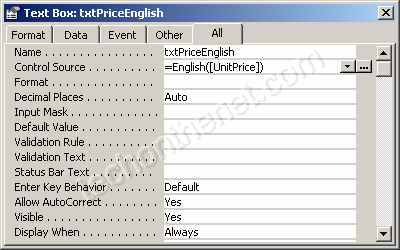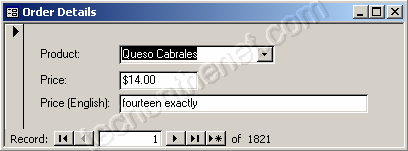## 8/06/2012

### Convert currency into words

Question: In Access, how can I convert currency to words?
For example,
\$100 should read as "one hundred exactly"

Answer: To convert currency into words, you'll need to open your Access database and create a new module.

Then paste into the new module the following two functions:
Function English(ByVal N As Currency) As String
Const Thousand = 1000@
Const Million = Thousand * Thousand
Const Billion = Thousand * Million
Const Trillion = Thousand * Billion
If (N = 0@) Then English = "zero": Exit Function
Dim Buf As String: If (N < 0@) Then Buf = "negative " Else Buf = ""
Dim Frac As Currency: Frac = Abs(N - Fix(N))
If (N < 0@ Or Frac <> 0@) Then N = Abs(Fix(N))
Dim AtLeastOne As Integer: AtLeastOne = N >= 1
If (N >= Trillion) Then
Debug.Print N
Buf = Buf & EnglishDigitGroup(Int(N / Trillion)) & " trillion"
N = N - Int(N / Trillion) * Trillion
If (N >= 1@) Then Buf = Buf & " "
End If
If (N >= Billion) Then
Debug.Print N
Buf = Buf & EnglishDigitGroup(Int(N / Billion)) & " billion"
N = N - Int(N / Billion) * Billion
If (N >= 1@) Then Buf = Buf & " "
End If
If (N >= Million) Then
Debug.Print N
Buf = Buf & EnglishDigitGroup(N \ Million) & " million"
N = N Mod Million
If (N >= 1@) Then Buf = Buf & " "
End If
If (N >= Thousand) Then
Debug.Print N
Buf = Buf & EnglishDigitGroup(N \ Thousand) & " thousand"
N = N Mod Thousand
If (N >= 1@) Then Buf = Buf & " "
End If
If (N >= 1@) Then
Debug.Print N
Buf = Buf & EnglishDigitGroup(N)
End If
If (Frac = 0@) Then
Buf = Buf & " exactly"
ElseIf (Int(Frac * 100@) = Frac * 100@) Then
If AtLeastOne Then Buf = Buf & " and "
Buf = Buf & Format\$(Frac * 100@, "00") & "/100"
Else
If AtLeastOne Then Buf = Buf & " and "
Buf = Buf & Format\$(Frac * 10000@, "0000") & "/10000"
End If
English = Buf
End Function

Private Function EnglishDigitGroup(ByVal N As Integer) As String
Const Hundred = " hundred"
Const One = "one"
Const Two = "two"
Const Three = "three"
Const Four = "four"
Const Five = "five"
Const Six = "six"
Const Seven = "seven"
Const Eight = "eight"
Const Nine = "nine"
Dim Buf As String: Buf = ""
Dim Flag As Integer: Flag = False
Select Case (N \ 100)
Case 0: Buf = "": Flag = False
Case 1: Buf = One & Hundred: Flag = True
Case 2: Buf = Two & Hundred: Flag = True
Case 3: Buf = Three & Hundred: Flag = True
Case 4: Buf = Four & Hundred: Flag = True
Case 5: Buf = Five & Hundred: Flag = True
Case 6: Buf = Six & Hundred: Flag = True
Case 7: Buf = Seven & Hundred: Flag = True
Case 8: Buf = Eight & Hundred: Flag = True
Case 9: Buf = Nine & Hundred: Flag = True
End Select
If (Flag <> False) Then N = N Mod 100
If (N > 0) Then
If (Flag <> False) Then Buf = Buf & " "
Else
EnglishDigitGroup = Buf
Exit Function
End If
Select Case (N \ 10)
Case 0, 1: Flag = False
Case 2: Buf = Buf & "twenty": Flag = True
Case 3: Buf = Buf & "thirty": Flag = True
Case 4: Buf = Buf & "forty": Flag = True
Case 5: Buf = Buf & "fifty": Flag = True
Case 6: Buf = Buf & "sixty": Flag = True
Case 7: Buf = Buf & "seventy": Flag = True
Case 8: Buf = Buf & "eighty": Flag = True
Case 9: Buf = Buf & "ninety": Flag = True
End Select
If (Flag <> False) Then N = N Mod 10
If (N > 0) Then
If (Flag <> False) Then Buf = Buf & "-"
Else
EnglishDigitGroup = Buf
Exit Function
End If
Select Case (N)
Case 0:
Case 1: Buf = Buf & One
Case 2: Buf = Buf & Two
Case 3: Buf = Buf & Three
Case 4: Buf = Buf & Four
Case 5: Buf = Buf & Five
Case 6: Buf = Buf & Six
Case 7: Buf = Buf & Seven
Case 8: Buf = Buf & Eight
Case 9: Buf = Buf & Nine
Case 10: Buf = Buf & "ten"
Case 11: Buf = Buf & "eleven"
Case 12: Buf = Buf & "twelve"
Case 13: Buf = Buf & "thirteen"
Case 14: Buf = Buf & "fourteen"
Case 15: Buf = Buf & "fifteen"
Case 16: Buf = Buf & "sixteen"
Case 17: Buf = Buf & "seventeen"
Case 18: Buf = Buf & "eighteen"
Case 19: Buf = Buf & "nineteen"
End Select
EnglishDigitGroup = Buf
End Function

Now, when you want to convert currency into words, you will reference the English function in your Access database as follows:
 English (100) would return "one hundred exactly" English (125) would return "one hundred twenty-five exactly" English (125.3) would return "one hundred twenty-five and 30/100"

Question: How do I get this function to work in a form?
Answer: To use this function, first copy the two functions listed above (English and EnglishDigitGroup) into a Module in your Access database.
Then, open your form in Design view, create a new text box, and view the properties for the new text box. Select the Control Source property and enter the following formula:
=English([UnitPrice])
What this formula does is display the English representation of the field UnitPrice.Now when you view your form, you should see the Unit Price information displayed in English in the last text box on the form.#### 1 comment:

1.Thanks for sharing the post.. parents are worlds best person in each lives of individual..they need or must succeed to sustain needs of the family. convert money online